# City Puzzle

Update 4/19/18: This puzzle was featured on FiveThirtyEight.com's Riddler column! Check it out.

I thought of a fun puzzle while I was at a conference in Downtown LA. You and I are in a city with a grid layout. All pedestrian signals alternate which direction is allowed to walk, and last exactly the same amount of time. To simplify, we are only allowed to cross one road at each intersection and we can never jaywalk.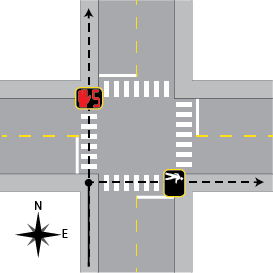We're trying to meet a friend at a restaurant one block north and two blocks east. We come to an intersection. We've got a walk signal going north, and a don't walk signal going east, with a big red timer counting down. The timer says one second. What do we do? Maybe you're thinking it doesn't matter?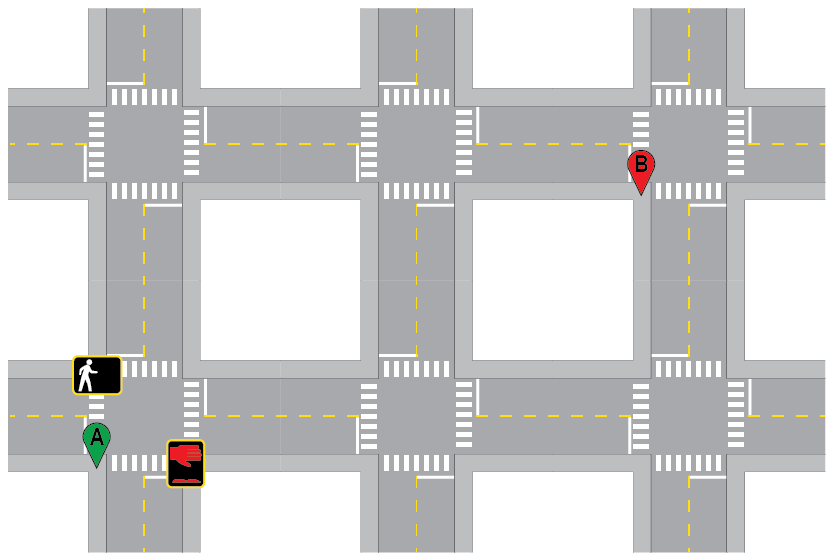Foolishly, you go north. Already, your fate is sealed. At the next intersection you can only go east, so you have no choice but to wait three seconds for the light to change. The intersection after that, you need to go east again. You wait an excruciating five seconds.

I go east. I lost one second waiting for the light to chance, but I'm not worried. As I arrive at the next intersection, I smile to myself and immediately walk whichever way the light allows, east in this case. At the next intersection, I wait a modest four seconds for the light to allow me to go north. One block later, I arrive at the intersection three precious seconds before you do. I high-five our mutual friend. You miss out.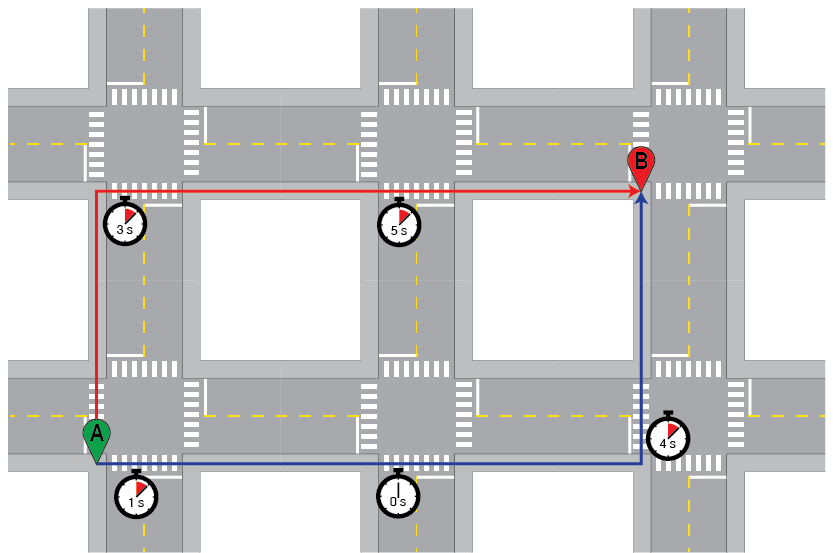What happened here? The only signal you know about is the one you're standing at. We know that you can go north immediately, but you have to wait one second to go east so, $$\tau_{north} = 0\text{ s}$$ and $$\tau_{east} = 1\text{ s}$$. We don't know anything about the other lights, but we can try to figure out some average behavior.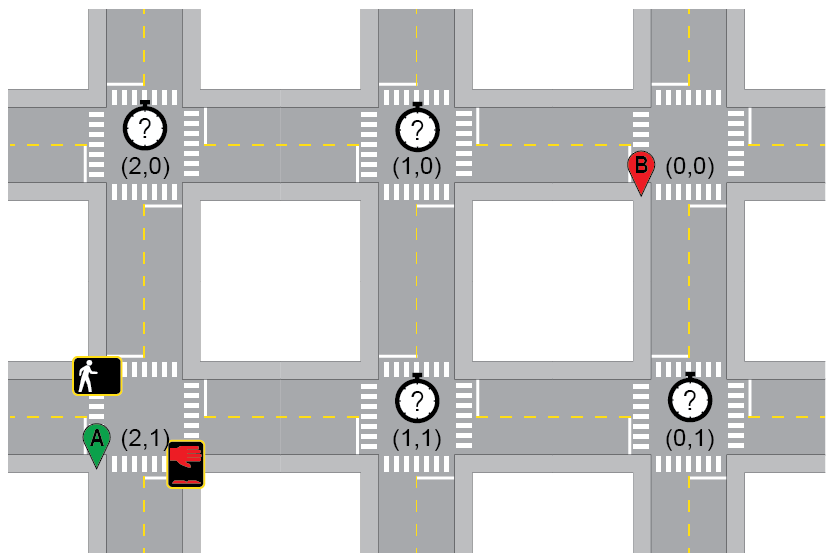To find the average wait time, let's plot out the system. Say each walk signal lasts a time $$T$$. The x-axis on the plot below is the time you arrive at the light, marked with the moments when the signals change. The y-axis is the resulting wait time to go in each direction. If you know nothing about the state of an intersection and you want to go east, you could get lucky and arrive when the light allows you to go east. You could also arrive and have to wait $$T$$ seconds. One in two chance of catching a walk signal. Average wait time of $$T/2$$ if you catch a don't walk signal. Overall, the average time you expect to wait is $$\left < t \right > = \frac{T}{4}$$.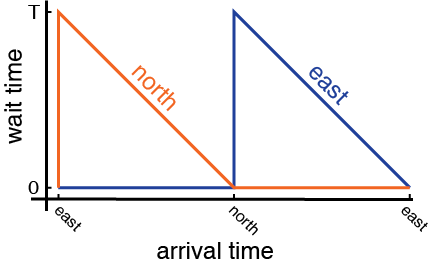We can apply this to the intersections in question. At the intersection (1,0), we must go east. We could get lucky and be able to go immediately, but we expect to wait an average of $$\frac{T}{4}$$. We'll write $$\left < t_{(1,0)} \right > = \frac{T}{4}$$. The situation at (0,1) is the same, $$\left < t_{(0,1)} \right > = \frac{T}{4}$$. At (2,0), you have to wait the same average time as at (1,0) twice, for a total wait time of $$\left < t_{(2,0)} \right > =2 \frac{T}{4}$$ from (2,0) to the finish line.

Things are different at (1,1). Here, you get to make a decision. You can immediately go to either (0,1) or (1,0), whichever the light allows. Because you get to choose, the intersection at (1,1) is free. That means a total wait time of only $$\left < t_{(1,1)} \right > = \frac{T}{4}$$ from here. That's the value of having multiple options.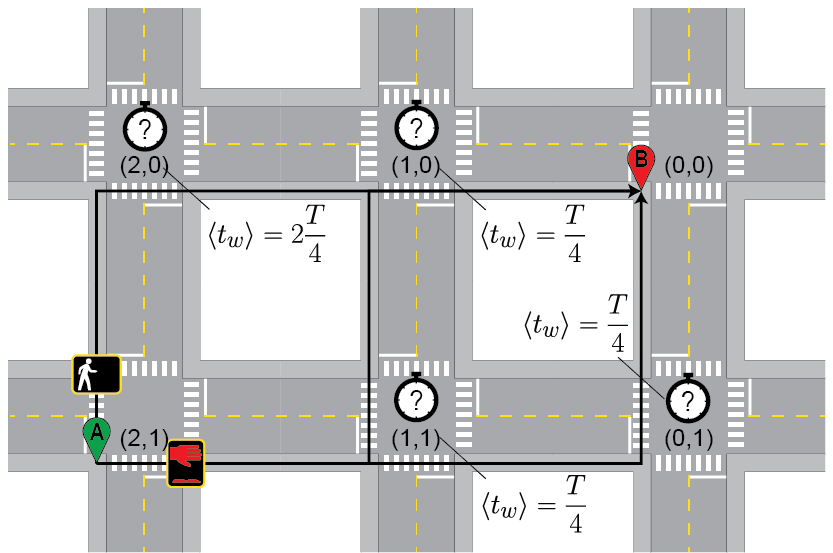The problem is almost solved. At (2,1), we know our options now. We can immediately go north to (2,0), where we expect to wait $$T/2$$, or we can wait one second and go to (1,1), where we expect to wait $$T/4$$. In other words, $$\left < t_{north}\right > = \frac{T}{2}$$ and $$\left < t_{east} \right > = \frac{T}{4} + 1\text{ s}$$. As long as T is longer than 4 seconds, you should go east. In general, you should go east unless $$\tau_{east} > \frac{T}{4}$$.

It's more complicated for other intersections, but I wrote some code to figure it out. If you're walking in an idealized city and you have Mathematica on your phone, feel free to use it to save time.